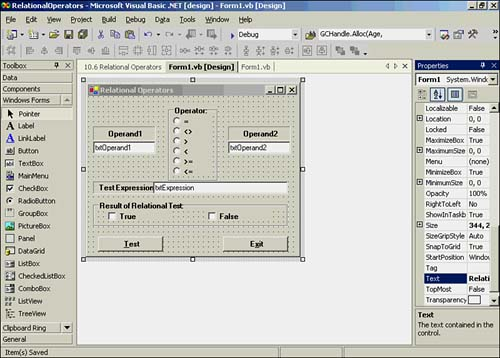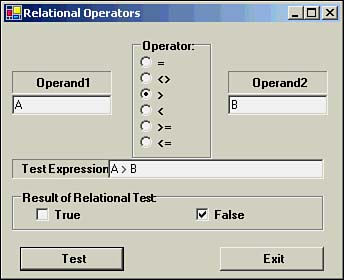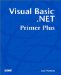# Relational Operators

The relational operators are used to compare values. These operators are presented in Table 10.1.

##### Table 10.1. Visual Basic .NET's Relational Operators

Relational Operator

Meaning

=

Equal

`  <>  `

Not equal

<

Less than

>

Greater than

`  <=  `

Less than or equal to

`  >=  `

Greater than or equal to

All the relational operators are binary operators. Recall from earlier chapters that a binary operator requires two operands. Therefore, the general syntax for the group of relational operators is as follows :

`  Operand1 RelationalOperator Operand2  `

When a statement uses a relational operator, the statement is said to be performing a relational test between two values (that is, operands). There are only two possible outcomes from a relational test: logic True or logic False .

#### A Simple If-Then-Else Statement

Relational tests are often performed by using If-Then-Else statements. Although you have used this statement in programs in earlier chapters, you have not learned its syntax. This is the syntax for the If-Then-Else statement:

` If  Expression1  Then    Execute if  Expression1  is True Else   Execute if  Expression1  is False End If `

The If statement block extends from the If keyword to the End If keywords. Expression1 is usually a relational test that either evaluates to logic True or logic False . If the relational test of Expression1 is logic True , the second line of the If statement is executed. If the relational test of Expression1 is logic False , the line following the Else keyword is executed.

Programmer's TipBy convention, the logic True and logic False statements of an If-Then-Else statement block are indented one tab stop. You will learn more about the If-Then-Else statement in Chapter 11, "Making Decisions."

Next, you can write a simple program that allows you to exercise both the If statement and the relational operators. Start a new project and call it RelationalOperators . Add the text boxes and buttons shown in Figure 10.1. (You could reuse the program you wrote for testing the arithmetic operators in Chapter 9, "Arithmetic and Assignment Operators," if you like.)

##### Figure 10.1. The form for the relational test program.Figure 10.1 shows a text box for each operand named txtOperand1 and txtOperand2 , and a third text box for the expression that is being tested . In other words, the text box named txtExpression presents the operands and operator that is called Expression1 in the syntax format for the If-Then-Else statement presented earlier. Near the bottom of the form in Figure 10.1 is a group box that holds two check boxes, which will show whether the relational test being performed is logic True or logic False .

The code for the program is simple. The operator group box contains six radio buttons, one for each of the relational operators. The code for the first radio button is presented in the following code fragment:

` Private Sub RadioButton1_CheckedChanged(ByVal sender As System.Object, _      ByVal e As System.EventArgs) Handles RadioButton1.CheckedChanged  WhichOne = 1 End Sub `

Whenever a radio button is clicked, the value of WhichOne is set to the selected radio button. WhichOne is an integer variable that is defined with module scope.

After the user types the two operands into his or her text boxes and selects the relational operator to test, he or she clicks the Test button. Listing 10.1 shows the complete code for the btnTest object's Click() event.

##### Listing 10.1 Code for the btnTest Object's Click Event
` Private Sub btnTest_Click(ByVal sender As System.Object, _              ByVal e As System.EventArgs) _           Handles btnTest.Click  ckbTrue.Checked = False   ' Set to false to begin with  ckbFalse.Checked = False  Select Case WhichOne   Case 1    txtExpression.Text = txtOperand1.Text & " = " & txtOperand2.Text    If txtOperand1.Text = txtOperand2.Text Then     ckbTrue.Checked = True    Else     ckbFalse.Checked = True    End If   Case 2    txtExpression.Text = txtOperand1.Text & " <> " & txtOperand2.Text    If txtOperand1.Text <> txtOperand2.Text Then     ckbTrue.Checked = True    Else     ckbFalse.Checked = True    End If   Case 3    txtExpression.Text = txtOperand1.Text & " > " & txtOperand2.Text    If txtOperand1.Text > txtOperand2.Text Then     ckbTrue.Checked = True    Else     ckbFalse.Checked = True    End If   Case 4    txtExpression.Text = txtOperand1.Text & " < " & txtOperand2.Text    If txtOperand1.Text < txtOperand2.Text Then     ckbTrue.Checked = True    Else     ckbFalse.Checked = True    End If   Case 5    txtExpression.Text = txtOperand1.Text & " >= " & txtOperand2.Text    If txtOperand1.Text >= txtOperand2.Text Then     ckbTrue.Checked = True    Else     ckbFalse.Checked = True    End If   Case 6    txtExpression.Text = txtOperand1.Text & " <= " & txtOperand2.Text    If txtOperand1.Text <= txtOperand2.Text Then     ckbTrue.Checked = True    Else     ckbFalse.Checked = True    End If  End Select End Sub `

The code in the procedure begins by setting the values of both of the check boxes to False . Then a Select Case statement is used to select the proper case to execute based on the value of WhichOne . In each Case of the Select statement, the txtExpression string is built by concatenating the operands in the txtOperand1 and txtOperand2 text boxes. The two operands are separated with a string literal that represents the relational operator being tested. A simple If test then determines whether to check the True check box or the False check box. All the Case statement blocks work in the same manner.

That's all there is to it. A sample run of the program is shown in Figure 10.2.

##### Figure 10.2. A sample run of the RelationalOperators program.The sample run in Figure 10.2 shows that you can also test string data with the relational operators. The example shows the test expression A > B is logic False . Why is the expression False ? Recall that string comparisons are made by using the numeric codes for the letters A and B . Because A equates to 65 and B is 66 , the expression A > B is False . Of course, you can test numeric values for the two operands, too.

You should experiment with the program so you become comfortable with how the relational operators work. You should use both numeric and string data for your experiments. Each string variable may contain more than a single character. For example, what happens if you test JOYCE against Joyce and why?Visual Basic .NET Primer Plus
ISBN: 0672324857
EAN: 2147483647
Year: 2003
Pages: 238
Authors: Jack Purdum

Similar book on Amazon